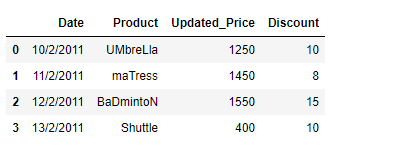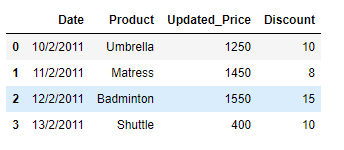Related Articles
Clean the string data in the given Pandas Dataframe
• Last Updated : 26 Jan, 2019

As we know, In today’s world data analytics is being used by all sorts of companies out there. While working with data, we can come across any sort of problem which requires an out of the box approach for evaluation. Most of the Data in real life contains the name of entities or other nouns. It might be possible that the names are not in proper format. In this post, we are going to discuss the approaches to clean such data.

Suppose we are dealing with the data of an e-commerce based website. The name of the products is not in the proper format. Properly format the data such that the there are no leading and trailing whitespaces as well as the first letters of all products are capital letter.

Solution #1: Many times we will come across a situation where we are required to write our own customized function suited for the task at hand.

 `# importing pandas as pd``import` `pandas as pd`` ` `# Create the dataframe``df ``=` `pd.DataFrame({``'Date'``:[``'10/2/2011'``, ``'11/2/2011'``, ``'12/2/2011'``, ``'13/2/2011'``],``                   ``'Product'``:[``' UMbreLla'``, ``'  maTress'``, ``'BaDmintoN '``, ``'Shuttle'``],``                   ``'Updated_Price'``:[``1250``, ``1450``, ``1550``, ``400``],``                   ``'Discount'``:[``10``, ``8``, ``15``, ``10``]})`` ` `# Print the dataframe``print``(df)`

Output :Now we will writer our own customized function to solve this problem.

 `def` `Format_data(df):``    ``# iterate over all the rows``    ``for` `i ``in` `range``(df.shape[``0``]):`` ` `        ``# reassign the values to the product column``        ``# we first strip the whitespaces using strip() function``        ``# then we capitalize the first letter using capitalize() function``        ``df.iat[i, ``1``]``=` `df.iat[i, ``1``].strip().capitalize()`` ` `# Let's call the function``Format_data(df)`` ` `# Print the Dataframe``print``(df)`

Output :Solution #2 : Now we will see a better and efficient approach using Pandas `DataFrame.apply()` function.

 `# importing pandas as pd``import` `pandas as pd`` ` `# Create the dataframe``df ``=` `pd.DataFrame({'``'Date'``:[``'10/2/2011'``, ``'11/2/2011'``, ``'12/2/2011'``, ``'13/2/2011'``],``                   ``'Product'``:[``' UMbreLla'``, ``'  maTress'``, ``'BaDmintoN '``, ``'Shuttle'``],``                   ``'Updated_Price'``:[``1250``, ``1450``, ``1550``, ``400``],``                   ``'Discount'``:[``10``, ``8``, ``15``, ``10``]})`` ` `# Print the dataframe``print``(df)`

Output :Let’s use the Pandas `DataFrame.apply()` function to format the Product names in the right format. Inside the Pandas `DataFrame.apply()` function we will use lambda function.

 `# Using the df.apply() function on product column``df[``'Product'``] ``=` `df[``'Product'``].``apply``(``lambda` `x : x.strip().capitalize())`` ` `# Print the Dataframe``print``(df)`

Output :Attention geek! Strengthen your foundations with the Python Programming Foundation Course and learn the basics.

To begin with, your interview preparations Enhance your Data Structures concepts with the Python DS Course.

My Personal Notes arrow_drop_up